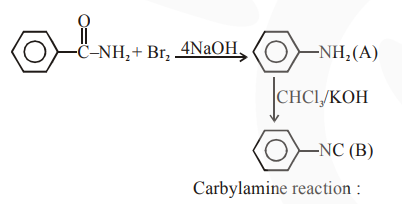# Hoffmann bromomide degradation of benzamide gives productQuestion:

Hoffmann bromomide degradation of benzamide gives product $A$, which upon heating with $\mathrm{CHCl}_{3}$ and $\mathrm{NaOH}$ gives product B. The structures of $\mathrm{A}$ and $\mathrm{B}$ are :

1.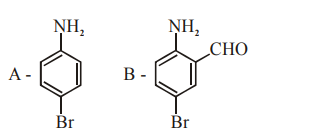2.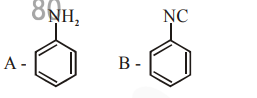3.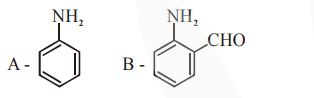4.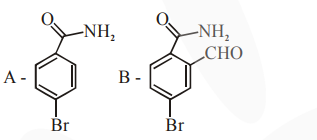Correct Option: , 2

Solution: